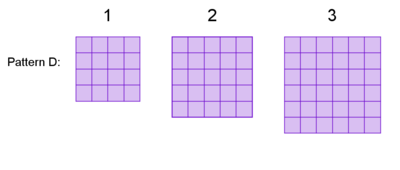##### Engage your students with effective distance learning resources. ACCESS RESOURCES>>

Alignments to Content Standards: A-REI.B.4.b

The first three steps of two visual patterns are shown below.The number of tiles in step $n$ of Pattern D is defined by $d(n)=(n+3)^2$. The number of tiles in step $n$ of Pattern E is defined by $e(n)=(n+1)^2-2$.

1. For each pattern, decide whether there is a step with 167 tiles in it. If so, which step is it? If not, explain how you know.
2. For each pattern, decide whether there is a step with 169 tiles in it. If so, which step is it? If not, explain how you know.
3. Describe and justify the steps for solving the following equation for $x$: $$a(x-h)^2=k$$

## IM Commentary

This task belongs to a series of three tasks that has students process a sequence of tile figures with the property that the $n$-th figure in the sequence has $f(n)$ tiles, for some quadratic function $f$. Students are asked to analyze the functions in the context of questions about the sequence of figures, a process which involves manipulating the quadratic expressions into different forms (identifying square roots at first, then completing the square in the third task.) With solving quadratics, there can be an impulse to put everything in standard form and just use the quadratic formula. However, seeing structure is emphasized in the standards because of how it connects and helps in understanding many foundational concepts, and these tasks develop the ability to see structure when working with quadratic expressions and equations.

That the domain of these quadratic functions is the set of positive integers provides an interesting wrinkle which students might not be used to thinking about in the setting of quadratic functions. The functions roughly increase in complexity through the three tasks, with the intent that the techniques learned in each will be used and expanded in the subsequent tasks. The other tasks in the sequence are Quadratic Sequence 1 and Quadratic Sequence 3.

Ideally, students will have had some experience working with visual patterns prior to this task. If not, students might benefit from additional questions to familiarize themselves with the idea. For example, they could be asked to "draw the next step" for one or more patterns. Or students could be asked to discuss how they "see" the pattern growing (here is a good primer on what that might look like.) Or, they could be asked to evaluate d(1) and explain its meaning in terms of the number of tiles in Pattern D.

The purpose of this task is to motivate solving a quadratic equation where the variable of interest, $n$ can be isolated by "undoing" operations. The equations in this particular task highlight algebraic moves that are useful when students learn to solve quadratic equations by completing the square.

In part (c), students have an opportunity to express regularity in repeated reasoning (MP.8) by applying these same "undoing" operations to an equation to isolate a variable of interest in terms of other variables.

This task was inspired by visualpatterns.org.

## Solution

1. We'll check if Pattern D has a step with 167 tiles by attempting to solve $$(n+3)^2=167.$$ But if this were true, 167 would need to be the square of $n+3$, and $167$ is not a perfect square. So Pattern D does not have a step with 167 tiles. To check if Pattern E has a step with 167 tiles, we'll attempt to solve $$(n+1)^2-2=167$$ $$(n+1)^2=169$$ $$n+1=13$$ $$n=12$$ (Note that we wrote $n+1=13$ instead of $n+1=\pm 13$ since $n$ has to be positive.)  Now since $e(12)=167$, we conclude that step $12$ of pattern E has $167$ tiles.
2. Checking Pattern D, we'll try to solve $$(n+3)^2=169$$ $$n+3=13$$ $$n=10$$ Since $d(10)=169$, step 10 of pattern D has 169 tiles. Checking $e(n)=169$, $$(n+1)^2-2=169$$ $$(n+1)^2=171$$ But since $171$ isn't a perfect square, $n+1$ is not an integer, so Pattern E does not have a step with $169$ tiles.
3. In parts (a) and (b), we solved equations of this form using a chain of reasoning. Here we'll do that generally. $$a(x-h)^2=k$$ $$(x-h)^2=\frac{k}{a}$$ $$x-h=\pm\sqrt{\frac{k}{a}}$$ $$x=h\pm\sqrt{\frac{k}{a}}$$